# How to Add Fractions with Unlike Denominators

Instructor: Laura Pennington

Laura received her Master's degree in Pure Mathematics from Michigan State University. She has 15 years of experience teaching collegiate mathematics at various institutions.

Let's look at how to add fractions with different denominators. After we have the solving process down, we will look at a few different examples showing how this can show up all the time in our daily lives.

## Steps to Solve

We want to add fractions with different denominators. To do this, it is good to know how to add fractions with common denominators. When two fractions have a common denominator, to add them together we simply add their numerators to get the numerator of the sum, and the denominator of the sum stays the same.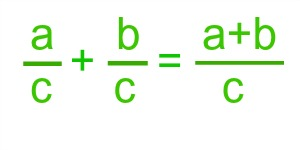To illustrate this, let's consider a pie, because everyone loves pie! If we cut the pie into 8 equal size pieces, then each piece is 1/8 of the pie. You eat two pieces of pie, so you ate (1/8) + (1/8) = 2/8, or 1/4, of the pie - delicious!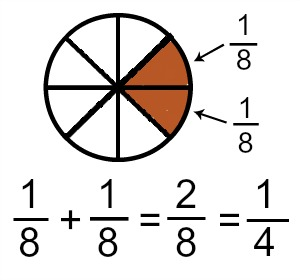Now suppose you ate 1 piece and wanted more, but not a whole piece, so you cut the other piece in half, creating a slice that is 1/16 of the pie. When this is the case, you ate 1/8 + 1/16 of the pie, but you can't just add the numerators since the slices are different sizes.

However, if we consider the slice that is 1/8 of the pie as 2/16 of the pie, then we see that we've eaten 2/16 + 1/16 = 3/16 of the pie.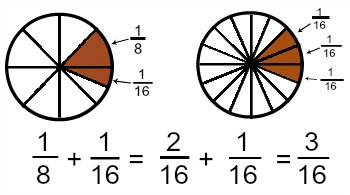When we are adding two fractions with different denominators, we should manipulate the fractions to find a common denominator, and then all we have to do is add the numerators. There are different ways to go about getting a common denominator on fractions. We are going to go with one of the easiest ways, and that is with a formula.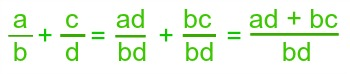Consider our pie example again. We know that 1/8 + 1/16 = 3/16. Let's use our formula to verify this.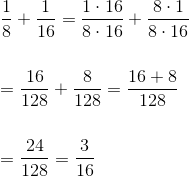We see that our formula also gives that 1/8 + 1/16 = 3/16.

## Solution

To add fractions with different denominators, we find a common denominator, and then we add the numerators as the following formula demonstrates.## Applications

Adding fractions comes up all the time in our daily lives. Let's look at a few different applications of adding fractions with different denominators in real life scenarios.

The first application we're going to look at has to do with distance. Suppose you are trying to get in shape, and you are tracking how many miles you walk or jog throughout the day. You know that when you walk to work from your car, it is a distance of 1/4 mile. Since you make that trip twice a day, this counts for 1/2 a mile of walking. When you get home from work, you end up having to park your car 5/8 of a mile from your house. By the time you get home, you tally up how much you have walked so far. That is, you want to add 1/2 + 5/8, so we use our formula.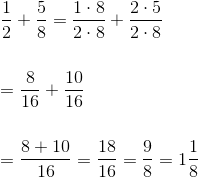Now you know that so far you've walked 9/8 of a mile, or 1 and 1/8 of a mile.

To unlock this lesson you must be a Study.com Member.

### Register to view this lesson

Are you a student or a teacher?

#### See for yourself why 30 million people use Study.com

##### Become a Study.com member and start learning now.
Back
What teachers are saying about Study.com

### Earning College Credit

Did you know… We have over 200 college courses that prepare you to earn credit by exam that is accepted by over 1,500 colleges and universities. You can test out of the first two years of college and save thousands off your degree. Anyone can earn credit-by-exam regardless of age or education level.